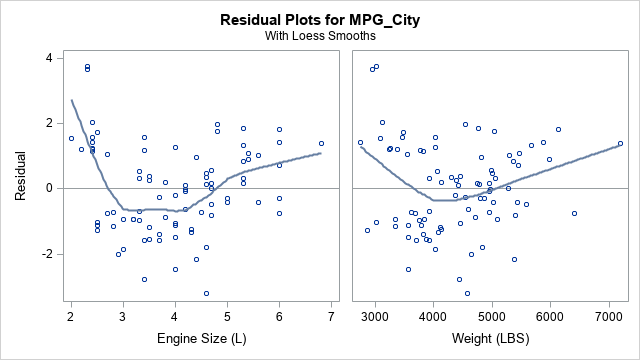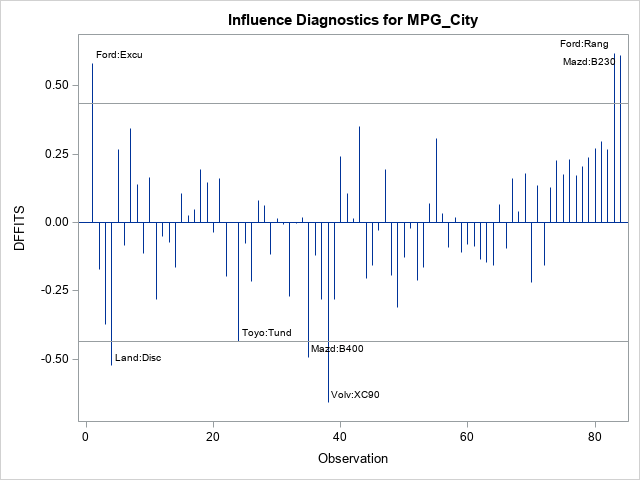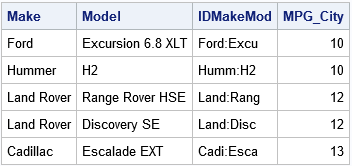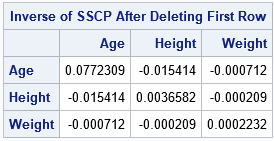# Posts Tagged ‘ Linear Regression ’

## Add loess smoothers to residual plots

June 24, 2019
ByWhen fitting a least squares regression model to data, it is often useful to create diagnostic plots of the residuals versus the explanatory variables. If the model fits the data well, the plots of the residuals should not display any patterns. Systematic patterns can indicate that you need to include

Tags: , , ,
Posted in SAS | Comments Off on Add loess smoothers to residual plots

## Influential observations in a linear regression model: The DFFITS and Cook’s D statistics

June 19, 2019
ByA previous article describes the DFBETAS statistics for detecting influential observations, where "influential" means that if you delete the observation and refit the model, the estimates for the regression coefficients change substantially. Of course, there are other statistics that you could use to measure influence. Two popular ones are the

Tags: , ,
Posted in SAS | Comments Off on Influential observations in a linear regression model: The DFFITS and Cook’s D statistics

## Influential observations in a linear regression model: The DFBETAS statistics

June 17, 2019
ByMy article about deletion diagnostics investigated how influential an observation is to a least squares regression model. In other words, if you delete the i_th observation and refit the model, what happens to the statistics for the model? SAS regression procedures provide many tables and graphs that enable you to

Tags: , ,
Posted in SAS | Comments Off on Influential observations in a linear regression model: The DFBETAS statistics

## Leave-one-out statistics and a formula to update a matrix inverse

June 12, 2019
ByFor linear regression models, there is a class of statistics that I call deletion diagnostics or leave-one-out statistics. These observation-wise statistics address the question, "If I delete the i_th observation and refit the model, what happens to the statistics for the model?" For example: The PRESS statistic is similar to